Next: References Up: Applications Previous: Stereo Camera Calibration

## Hough Transform SEM Calibration

The standard set of parameters required to define a CCD camera are;

• focal length (f)
• image centres (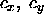)
• aspect ratios (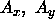)

In order to define a stereo camera system two such sets of parameters are required (one for each camera L and R) together with additional parameters describing the between camera transformation which is best described using a minimum of six degrees of freedom. For this we use the last three parameters of the quaternion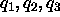where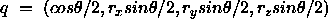describing the camera rotation, ( the last free parameter is recovered from the normalisation constraint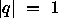), plus the interoccular separation I plus two components of the translation direction vector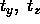.

However, the relatively simple geometric behaviour of an SEM stereo system makes it possible to describe the allowable epi-polar geometry in terms of a small number of free parameters:

• relative image centres (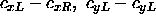).
• affine image rotation (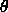).
• focal ratio (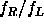).
For approximate orthographic projection the absolute image centres are in most respects arbitrary and can be taken as the centres of the matched image regions without any loss of performance. Moreover, the relative x coordinates are irrelevant with respect to the epi-polar constraint and can thus be fixed at default values. Also, for stereo samples adjusted for approximate equal sample depth the focal length ratio can be assumed to be 1.0 to within a few percent. An approximate epi-polar geometry can thus be constructed in which the only free parameters are the relative y shift of the images and an affine rotation.

Using a two-dimensional Hough technique, candidate matches from a stereo corner matcher (Figure 11a) can be used to identify the remaining degrees of freedom (Figure 10). The final set of matches which are consistent with this solution are shown in Figure 11b.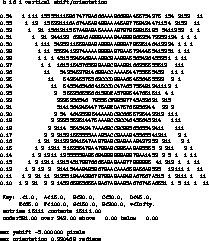Figure 10: Output from the Hough matcher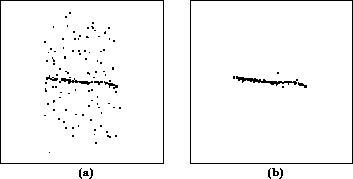Figure 11: Reprojected candidate matches a) before b) after hough filtering

Acknowledgements

The authors would like to thank A.Hill, A.Brett and J.Schumm for their help with the LaTeX.Next: References Up: Applications Previous: Stereo Camera Calibration

Bob Fisher
Fri Mar 28 14:12:50 GMT 1997